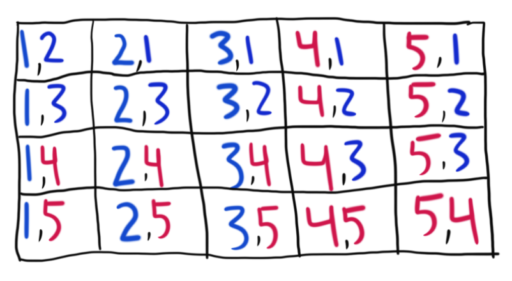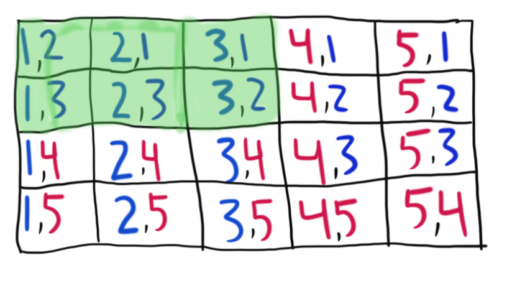So far we have talked about calculating probabilities of events where we tell you all the possible outcomes. For example, when we flip a coin, the possible outcomes are known: heads or tails. In such examples, you know how many possible outcomes there are. If we roll a six-sided die, there are clearly just six outcomes. But what if we are not given the number of possible outcomes? Consider this problem:

Example
There is a bag of 5 marbles; 3 blue and 2 red. Jane picks one marble out of the bag, looks at its color, sets it to the side (and does not return it to the bag) and picks another marble. What is the probability that she picks two blue marbles?

In this case, it isn’t clear how to apply our earlier rules. Remember our first rule:

Probability of Equally Likely Outcomes:
If you have n possible outcomes, all of which are equally likely, then the probability of any particular outcome occurring is 1/n.

How do we apply this rule to Jane’s situation? How many possible outcomes do we have?

This is where combinatorics comes in: it will allow us to determine how many possible outcomes we have. Now the word “combinatorics” is a little intimidating, but it’s just a fancy word for the mathematics of counting. In this case, we will use it to count the number of possible outcomes for Jane.

Let’s try to list out all the possible pairs of marbles that Jane could pick. To do this, let’s number our blue marbles 1 through 3, and number our red marbles 4 through 5. Now, Jane has to pick two different marbles. So, in other words, Jane ends up with some pair of marbles from the following list:So these are all the possible outcomes Jane could get. She could pick any of the 5 marbles first, and then she could pick any of the 4 remaining marbles second. Now, the question doesn’t say this explicitly, but we will assume (and it is generally fair game to assume) that Jane is equally likely to pick any of the marbles from the bag. After all, the marbles are presumably the same size and feel the same, so she’s just as likely to pick one as to pick any other.

Now, we have our set of equally likely outcomes. And these are all the outcomes. So, in line with our above rule, to find P(Jane picks 2 blue marbles) now we just need to pick out the outcomes where Jane picks two blue marbles. And that’s easy enough to see on our table, we just need to pick the outcomes where two blue marbles are chosen.Adding up these outcomes, we get 6/20 = 3/10. So that’s the likelihood of Jane picking two blue marbles.

In general, it will not be practical to make our table of possibilities and add things up. For if Jane had a bag of 10 marbles, we would have had 90 possible outcomes (10 choices for the first marble multiplied by 9 choices for the second). So, we introduce the following rule:

Rule for Permutations
Suppose we have n objects that we want to fill k spots where kn. Then, the number of possible ways ("permutations") to do this is:The n! is read as “n factorial” and it means n * (n-1) * (n-2) * … * (1). Basically, we take n and we multiply it by all the positive numbers less than or equal to it. Here are some examples:And, by a special convention, we say that 0! = 1.

Now, to solve probability questions, we want to find: how many permutations (or possible outcomes) will satisfy our event, and how many permutations in total are possible (i.e. how many possible outcomes there are). So in our above problem, we want to find:

1. How many permutations involve two blue marbles being drawn? (How many outcomes involve two blue marbles being drawn?)
We have three blue marbles, and we are drawing two marbles. So we want to use three objects to fill two spaces. Following our rule, we get:2. How many total permutations are there? (How many possible outcomes are there in total?)
We have five total marbles and we are drawing two of them. So we want to use five objects to fill two spaces. Following our rule, we get:Thus, we get: P(Draw two blue marbles) =, which matches our previous answer.

Practice Problems

Question 1
Suppose you have three friends: Anne, Beth, and Charles. How many ways can they form a three-person line?

Question 2
Mark is trying to descramble a series of letters: AQCFXDE. How many possible five-letter combinations can be formed from those letters?

Question 3
Jane is drawing four cards from a standard 52-card deck. What is the probability that she first draws the King of Hearts, and then the Ace of Spaces, and then the Jack of Clubs, and finally the Three of Hearts?